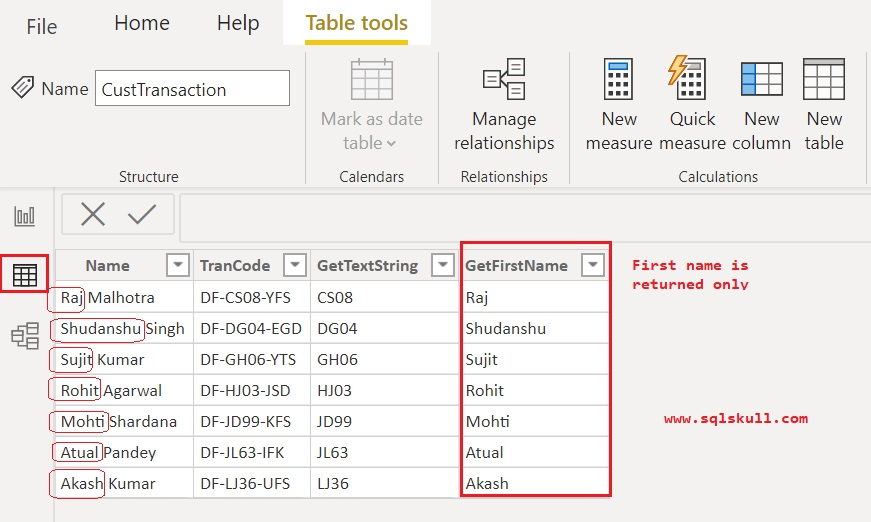Home » MID Function DAX

# MID Function DAXMID function is a Power BI text functions in DAX, it returns a string of characters from the middle of a text string based on a given starting position and length.

SYNTAX

`MID(<text>, <start_num>, <num_chars>)`

text  is the text string from which you want to extract the characters, or a column that contains text.

start_num is the position of the first character you want to extract. Positions start at 1.

num_chars is the number of characters to return.

Lets look at an example of using MID function in Power BI.

Here we have a sample data named CustTransaction as shown below.##### Copy Sample Data:
 Name TranCode Raj Malhotra DF-CS08-YFS Shudanshu Singh DF-DG04-EGD Sujit Kumar DF-GH06-YTS Rohit Agarwal DF-HJ03-JSD Mohti Shardana DF-JD99-KFS Atual Pandey DF-JL63-IFK Akash Kumar DF-LJ36-UFS

#### Using MID function

Assume that, you want to extract some specific text from the middle of string that column TranCode contains.

For example, a string DF-CS08-YFS in column TranCode, you want to extract text string CS08 that is between two hyphen(-).

To extract the text string that is between two hyphen there is a trick, you can see the first hyphen occurs at position 3rd so the position of the first character of text string that you want to extract will be 4.

And you also know that the text string that you want to extract have the same length for all records in table CustTransaction that is 4, as between two hyphen all the text string in column TranCode have same length.

So the start position of text string will be 4 and the length of text string that is number of character to be returned from text string will also be 4.

So the second argument start_num value will be 4 and the third argument value num_chars will be 4.

Lets create a calculated columns that uses the MID function and returns a specific text string from the middle of text string.

`GetTextString = MID(CustTransaction[TranCode], 4, 4)`Once you create the column, lets quickly see the output in Data view pane.

Go to Data view pane, there you can see the preview of data.You can see it returns the 4 characters from a specified position 4 that is the position of first character in text string.

Lets take a look at one more example. Assume that, you want to extract first name only, that can also extracted using MID function with the help of another DAX function FIND DAX function, which returns the starting position of one text string within another text string.

As you can see, a full name of customer’s combine the first name and last name which are separated by character space (” “). So using the FIND function, we will get the position of first occurrence of character space (” “) in string, which is used inside the MID function as a third argument num_chars  with subtracting -1, which will return the number of characters from string before the occurrence of space character.

Lets create one more new column named GetFirstName, that will return the first name value.

```GetFirstName =

MID ( CustTransaction[Name], 1, FIND ( " ", CustTransaction[Name], 1 ) -1)```You can see, the second argument start_num value is 1 that is the position of the first character of string as you want to extract first name, then the third argument num_chars value that are returned by FIND function after subtracting -1 will be the position of last character of first name, so that all the characters could be returned from string before the occurrence of space character.

Lets see the output of column in Data view pane.You can see, it returns the first name of customers only.